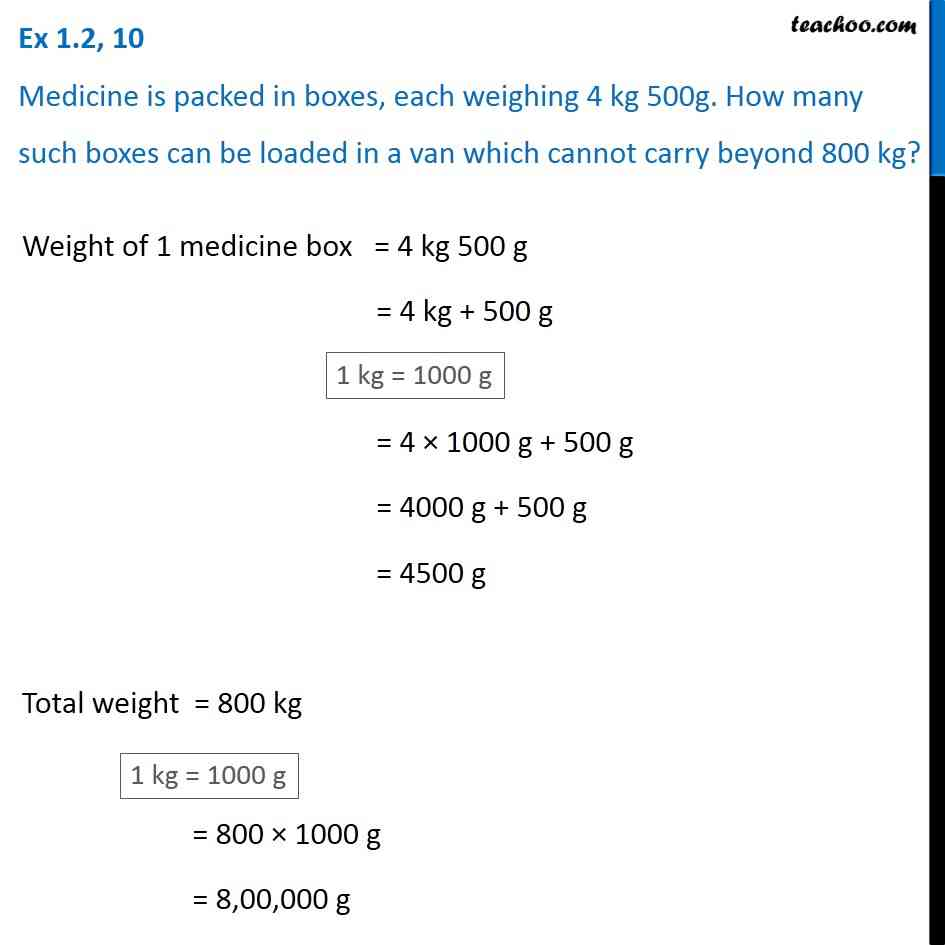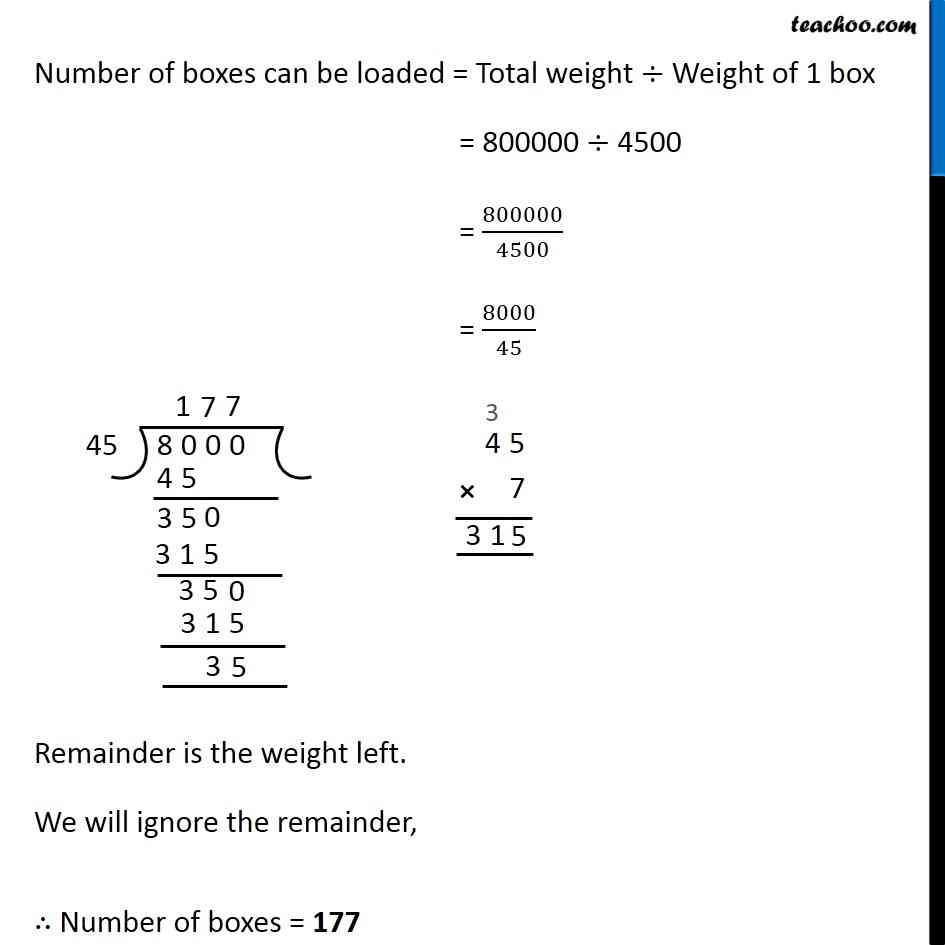1. Chapter 1 Class 6 Knowing our Numbers
2. Concept wise
3. Division of large numbers

Transcript

Ex 1.2, 10 Medicine is packed in boxes, each weighing 4 kg 500g. How many such boxes can be loaded in a van which cannot carry beyond 800 kg?Weight of 1 medicine box = 4 kg 500 g = 4 kg + 500 g = 4 1000 g + 500 g = 4000 g + 500 g = 4500 g Total weight = 800 kg = 800 1000 g = 8,00,000 g Number of boxes can be loaded = Total weight Weight of 1 box = 800000 4500 = 800000/4500 = 8000/45 Remainder is the weight left. We will ignore the remainder, Number of boxes = 177

Division of large numbers